# Comparing positive and negative numbers on the number line

##By Khan Academy

Comparing positive and negative numbers on the number line# Negative numbers on the number line without reference to zero

##By Khan Academy

Given a few negative numbers on a number line, let's see if we can determine what negative numbers are elsewhere on the number line.# Multiplying and dividing negative numbers

##By Khan Academy

Use the distributive property to understand the products of negative numbers.# Imaginary roots of negative numbers | Imaginary and complex numbers | Precalculus | Khan Academy

##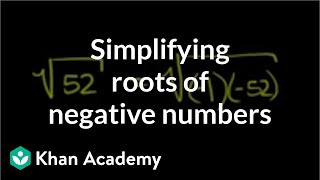By Khan Academy

Khan Academy presents Imaginary Roots of Negative Numbers, an educational video resource on math.# Interpreting negative numbers

##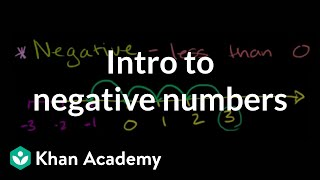By Khan Academy

Mysterious negative numbers! What ARE they? They are numbers less than zero. If you understand the nature of below zero temperatures, you can understand negative numbers. We'll help.# Multiplying and dividing negative numbers

##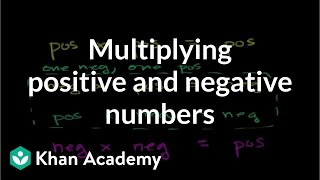By Khan Academy

Learn some rules of thumb for multiplying positive and negative numbers.# Multiplying and dividing negative numbers

##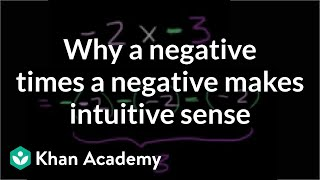By Khan Academy

Use the repeated addition model of multiplication to give an understanding of multiplying negative numbers.# Ordering negative numbers

##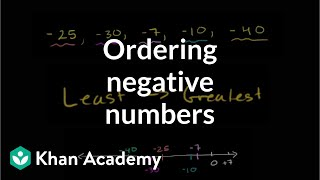By Khan Academy

Is -40 bigger than -10? When ordering negative numbers from least to greatest, careful that you don't get hung up on the amount of the number. Think about what that negative sign really means!# Subtracting negative numbers intro

##By Khan Academy

Find out why subtracting a negative number is the same as adding the absolute value of that number.# Negative numbers on the number line

##By Khan Academy

Mysterious negative numbers! What ARE they? They are numbers less than zero. If you understand the nature of below zero temperatures, you can understand negative numbers. We'll help.# Negative numbers on the number line

##By Khan Academy

Mysterious negative numbers! What ARE they? They are numbers less than zero. If you understand the nature of below zero temperatures, you can understand negative numbers. We'll help.# Negative numbers on the number line

##By Khan Academy

Mysterious negative numbers! What ARE they? They are numbers less than zero. If you understand the nature of below zero temperatures, you can understand negative numbers. We'll help.# Negative numbers on the number line

##By Khan Academy

Mysterious negative numbers! What ARE they? They are numbers less than zero. If you understand the nature of below zero temperatures, you can understand negative numbers. We'll help.# Comparing positive and negative numbers on the number line

##By Khan Academy

Comparing positive and negative numbers on the number line# Comparing positive and negative numbers on the number line

##By Khan Academy

Comparing positive and negative numbers on the number line# Comparing positive and negative numbers on the number line

##By Khan Academy

Comparing positive and negative numbers on the number line# Comparing positive and negative numbers on the number line

##By Khan Academy

Comparing positive and negative numbers on the number line# Negative numbers on the number line without reference to zero

##By Khan Academy

Given a few negative numbers on a number line, let's see if we can determine what negative numbers are elsewhere on the number line.# Negative numbers on the number line without reference to zero

##By Khan Academy

Given a few negative numbers on a number line, let's see if we can determine what negative numbers are elsewhere on the number line.# Negative numbers on the number line without reference to zero

##By Khan Academy

Given a few negative numbers on a number line, let's see if we can determine what negative numbers are elsewhere on the number line.